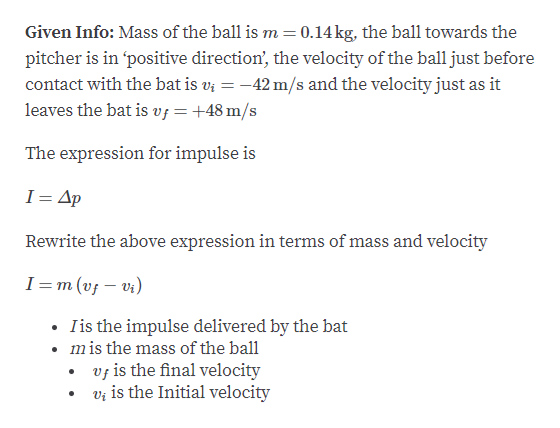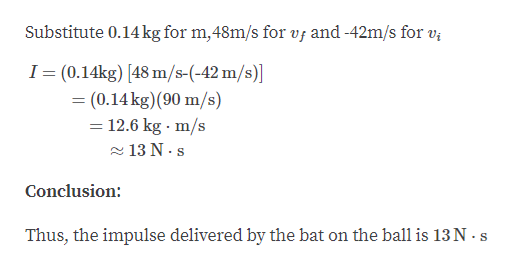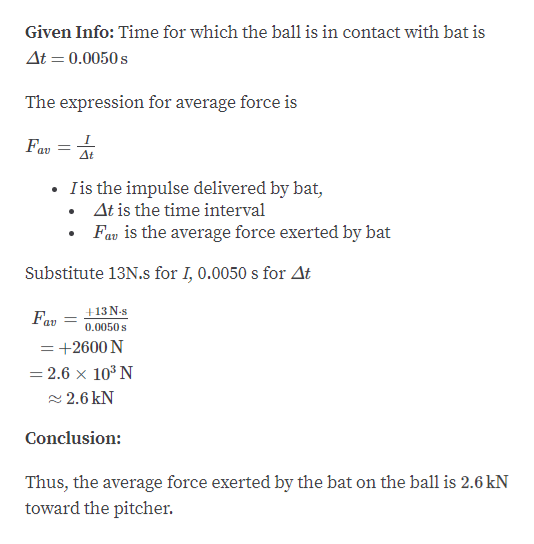# A pitcher throws a 0.14-kg baseball toward the batter sothat it crosses home plate horizontally and has a speed of 42 m/sjust before it makes contact with the bat. The batter then hits theball straight back at the pitcher with a speed of 48 m/s. Assumethe ball travels along the same line leaving the bat as it followedbefore contacting the bat. (a) What is the magnitude of theimpulse delivered by the bat to the baseball? (b) If the ball is incontact with the bat for 0.005 0 s, what is the magnitude of theaverage force exerted by the bat on the ball? (c) How does youranswer to part (b) compare to the weight of the ball?

Question
7 views

A pitcher throws a 0.14-kg baseball toward the batter so
that it crosses home plate horizontally and has a speed of 42 m/s
just before it makes contact with the bat. The batter then hits the
ball straight back at the pitcher with a speed of 48 m/s. Assume
the ball travels along the same line leaving the bat as it followed
before contacting the bat. (a) What is the magnitude of the
impulse delivered by the bat to the baseball? (b) If the ball is in
contact with the bat for 0.005 0 s, what is the magnitude of the
average force exerted by the bat on the ball? (c) How does your
answer to part (b) compare to the weight of the ball?

check_circle

Step 1

Part A:help_outlineImage TranscriptioncloseGiven Info: Mass of the ball is m=0.14kg, the ball towards the pitcher is in 'positive direction', the velocity of the ball just before contact with the bat is v; = -42 m/s and the velocity just as it leaves the bat is v¡ =+48 m/s The expression for impulse is I= Ap Rewrite the above expression in terms of mass and velocity I =m (vf – vi) • Iis the impulse delivered by the bat mis the mass of the ball v; is the final velocity vị is the Initial velocity fullscreen
Step 2help_outlineImage TranscriptioncloseSubstitute 0.14 kg for m,48m/s for vf and -42m/s for v; I= (0.14kg) [48 m/s-(-42 m/s)] = (0.14 kg)(90 m/s) = 12.6 kg · m/s - 13 N · s Conclusion: Thus, the impulse delivered by the bat on the ball is 13 N - s fullscreen
Step 3

part B:...help_outlineImage TranscriptioncloseGiven Info: Time for which the ball is in contact with bat is At = 0.0050 s The expression for average force is Fav = At Iis the impulse delivered by bat, • At is the time interval Fav is the average force exerted by bat Substitute 13N.s for I, 0.0050 s for At +13N-s Fav %3D 0.0050 s = +2600 N = 2.6 x 10³ N - 2.6 kN Conclusion: Thus, the average force exerted by the bat on the ball is 2.6 kN toward the pitcher. fullscreen

### Want to see the full answer?

See Solution

#### Want to see this answer and more?

Solutions are written by subject experts who are available 24/7. Questions are typically answered within 1 hour.*

See Solution
*Response times may vary by subject and question.
Tagged in

### Collisions